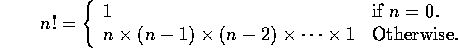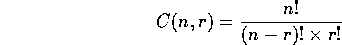# Functions with Parameters

Tom Kelliher, CS17

Mar. 25, 1996

```int add(int a, int b);

int main()
{
int i = 12;
int j = 4;
int k;

cout << k << endl;

return 0;
}

{
return a + b;
}
```

• Calling, called function.
• Calling functions communicates through parameters.
• Called function communicates through return value.
• Don't use global variables for communication.

# Parameter Passing by Value

## An Example

Consider:

```void f(void);
void changeMe(int);

void f(void)
{
int a = 5;
int i = 2;

changeMe(4);

changeMe(a);
cout << a << endl;

changeMe(i);
cout << i << endl;

changeMe(i + 4);
}

void changeMe(int a)
{
a = 0;
}
```

Is changeMe(4); legal?

What's printed?

## Some Nomenclature

• A Formal parameter:
• Function declaration, definition.
• Always a variable.
• Local variable of function.
• Local scope, visibility.
• An Actual parameters
• Function call.
• Any expression of appropriate type.
• Must match in lists.

Semantics of a function call:

1. Storage allocated for the formal parameters.
2. Actual arguments evaluated within calling function.
3. Their values used to initialize formal parameters.
4. Called function runs.
5. Storage for formal parameters de-allocated when called function returns.

# Modular Program Design Problems

1. Design a modular program which computes factorials of non-negative integers:The program should accept positive integers from the user, asking them if they want to continue, and output the factorial of the input.

2. Using modules from the previous program, write a modular program which computes combinations. The formula is:C(n,r) is the number of ways in which r objects can be drawn from a set of n objects, assuming that order doesn't matter. The user interface of this program should be similar to the previous one. I.e., accept input for several computations, ask the user if they want to continue, etc.

Thomas P. Kelliher
Thu Mar 21 13:29:29 EST 1996
Tom Kelliher